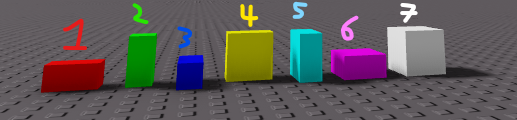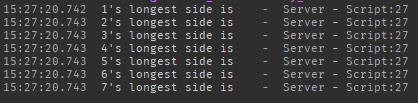# Optimizing a function to find the longest side(s) of a part

Whilst developing mechanics for my upcoming Scrapyard game, I had this interesting thought where, as you salvage materials from wreckage, the parts that make up the trash model gradually shrink to nothingness until, when the Model has no base parts left inside, it gets deleted.

So, to add to this concept, I developed a `function findLongest(part)`.

## What does the code do?

The function does this:

``````function findLongest(part)
local s = part.Size			--	Get the part's size.
local x = s.X
local y = s.Y
local z = s.Z

if x>y and x>z then			--	X is longer than Y and Z
return "X"
elseif y>x and y>z then		--	Y is longer than Z and X
return "Y"
elseif z>x and z>y then		--	Z is longer than X and Y
return "Z"
elseif x==y and y>z then	--	Z is shorter than X and Y; X:Y
return "XY"
elseif y==z and z>x then	--	X is shorter than Y and Z; Y:Z
return "YZ"
elseif z==x and x>y then	--	Y is shorter than Z and X; Z:X
return "XZ"
else						--	X == Y == Z
return "XYZ"
end
end
``````
• If all of a part’s sides are not equal to each other, it will return the longest of the sides.
• If two of the sides are equal to each other and longest of them all, it will return these two sides.
• If these two last statements are false, then this means the object is equilateral, so `return "XYZ"`.

FindLongestDemo.rbxl (23.5 KB)
This .rbxl file demonstrates the function.

## What are you not satisfied with?

I am not sure if the code is efficient enough with the long `if-elseif-else` chain.

## What potential improvements have you considered?

So, I was thinking, “Could I do something else that is better than a long `if-elseif-else` chain?”

## How (specifically) do you want to improve the code?

I am trying to optimize the function by finding a better alternative to a long `if-elseif-else` chain; if you have a better method than the chain, please notify me. Otherwise, if the code is fine enough, you could say that too.

Use math.max instead. Local max = math.max(Size.X, Size.Y, Size.Z)

4 Likes

@rotbotrotbot gave a great example that I would like to extend a bit.

``````local axisNames = {"X", "Y", "Z"}

local maxAxisNames = {}
local maxSize = math.max(size.X, size.Y, size.Z)

for _, axisName in pairs(axisNames) do
if maxSize == size[axisName] then
table.insert(maxAxisNames, axisName)
end
end

result = table.concat(maxAxisNames, "")
``````

EDIT: Fix small issue, you probably seen

1 Like

It’s… good. I’ll try that out!

1 Like

There is a problem with what you suggested.

`result` is `nil`. I added to the function:

``````--	Let's say that the dimensions of the part are 2,1,1

function findLongest(part)
local axisNames = {"X", "Y", "Z"}
local size = part.Size
local X = size.X
local Y = size.Y
local Z = size.Z
print(X,Y,Z)	-- 2,1,1

local maxAxisNames = {}
local maxSize = math.max(X,Y,Z)
print(maxSize)	-- 2

for _,v	 in ipairs(axisNames) do
if maxSize == v then
table.insert(maxAxisNames,v)
end
end

local result = table.concat(maxAxisNames, "")
return result
end
``````

Applying the function, I set up a model, `M`, that looks like this:I am facing +Z.

1. `2,1,1`
2. `1,2,1`
3. `1,1,2`
4. `2,2,1`
5. `1,2,2`
6. `2,1,2`
7. `2,2,2`

I ran the function like so:

``````M = game.Workspace.Model

for _,v in ipairs(M:GetChildren()) do
local l = findLongest(v)
print(v.Name.."'s longest side is ",l)
end
``````

However, the results show that `findLongest(v)` returns a blank space. What is wrong?It is supposed to print out “#'s longest side is XYZ” or something…

Yes, I have edited my reply that day to fix the issue

1 Like